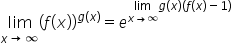Maths-
General
Easy

###Hint:

To solve the given function, we will use the formulaafter using the formula we will solve the function till that stage when putting the value of x will not make the function undefined, later we will put the value of x and get the required answer.

## Book A Free Demo+91